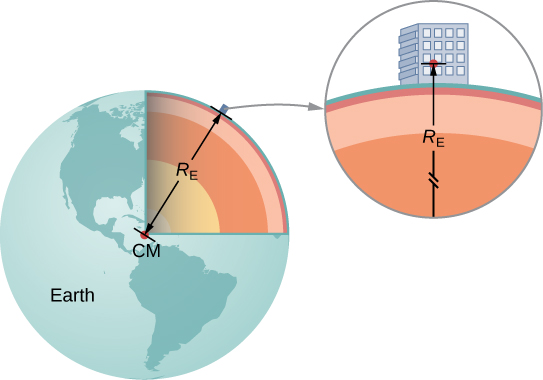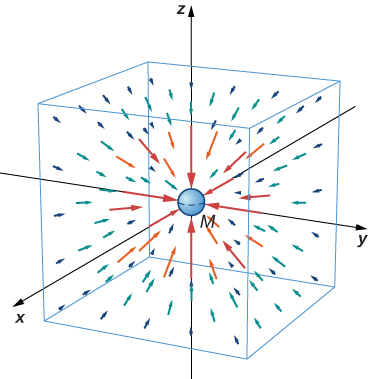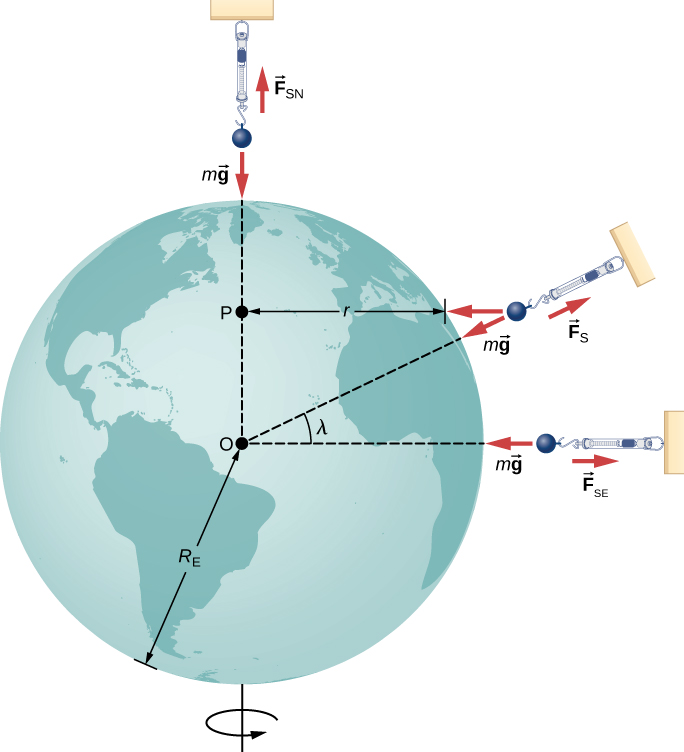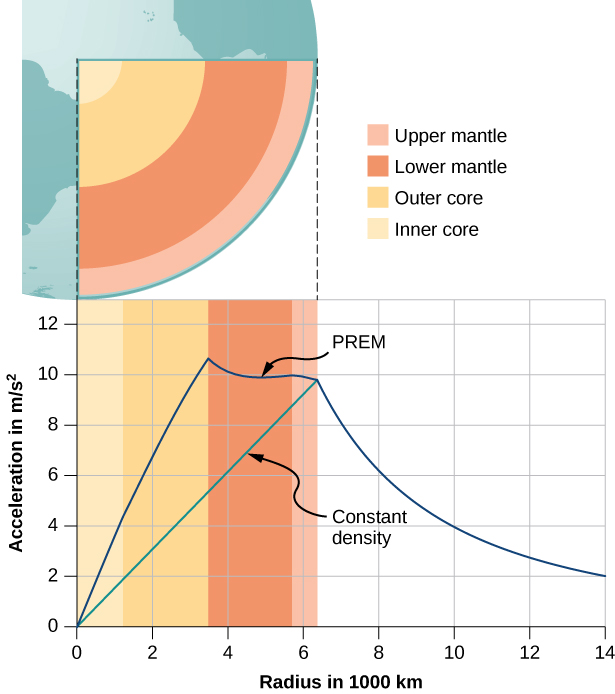## 13.2 Gravitation Near Earth’s Surface

### Learning Objectives

By the end of this section, you will be able to:

• Explain the connection between the constants $G$ and $g$
• Determine the mass of an astronomical body from free-fall acceleration at its surface
• Describe how the value of $g$ varies due to location and Earth’s rotation

In this section, we observe how Newton’s law of gravitation applies at the surface of a planet and how it connects with what we learned earlier about free fall. We also examine the gravitational effects within spherical bodies.

### Weight

Recall that the acceleration of a free-falling object near Earth’s surface is approximately $g=9.80\,{\text{m/s}}^{2}$. The force causing this acceleration is called the weight of the object, and from Newton’s second law, it has the value mg. This weight is present regardless of whether the object is in free fall. We now know that this force is the gravitational force between the object and Earth. If we substitute mg for the magnitude of ${\overset{\to }{F}}_{12}$ in Newton’s law of universal gravitation, m for ${m}_{1}$, and ${M}_{\text{E}}$ for ${m}_{2}$, we obtain the scalar equation

$mg=G\,\frac{m{M}_{\text{E}}}{{r}^{2}}$

where r is the distance between the centers of mass of the object and Earth. The average radius of Earth is about 6370 km. Hence, for objects within a few kilometers of Earth’s surface, we can take $r={R}_{\text{E}}$ ((Figure)). The mass m of the object cancels, leaving

$g=G\frac{{M}_{\text{E}}}{{r}^{2}}.$

This explains why all masses free fall with the same acceleration. We have ignored the fact that Earth also accelerates toward the falling object, but that is acceptable as long as the mass of Earth is much larger than that of the object.Figure 13.7 We can take the distance between the centers of mass of Earth and an object on its surface to be the radius of Earth, provided that its size is much less than the radius of Earth.

### Example

#### Masses of Earth and Moon

Have you ever wondered how we know the mass of Earth? We certainly can’t place it on a scale. The values of g and the radius of Earth were measured with reasonable accuracy centuries ago.

1. Use the standard values of g, ${R}_{\text{E}}$, and (Figure) to find the mass of Earth.
2. Estimate the value of g on the Moon. Use the fact that the Moon has a radius of about 1700 km (a value of this accuracy was determined many centuries ago) and assume it has the same average density as Earth, $5500\,{\text{kg/m}}^{3}$.

#### Strategy

With the known values of g and ${R}_{\text{E}}$, we can use (Figure) to find ${M}_{\text{E}}$. For the Moon, we use the assumption of equal average density to determine the mass from a ratio of the volumes of Earth and the Moon.

#### Solution

1. Rearranging (Figure), we have
${M}_{\text{E}}=\frac{g{R}_{\text{E}}^{2}}{G}=\frac{9.80\,{\text{m/s}}^{2}{(6.37\,×\,{10}^{6}\,\text{m})}^{2}}{6.67\,×\,{10}^{-11}\,\text{N}·{\text{m}}^{2}{\text{/kg}}^{2}}=5.95\,×\,{10}^{24}\,\text{kg.}$
2. The volume of a sphere is proportional to the radius cubed, so a simple ratio gives us
$\frac{{M}_{\text{M}}}{{M}_{\text{E}}}=\frac{{R}_{\text{M}}^{3}}{{R}_{\text{E}}^{3}}\to {M}_{\text{M}}=(\frac{{(1.7\,×\,{10}^{6}\,\text{m})}^{3}}{{(6.37\,×\,{10}^{6}\,\text{m})}^{3}})(5.95\,×\,{10}^{24}\,\text{kg})=1.1\,×\,{10}^{23}\,\text{kg.}$

We now use (Figure).

${g}_{\text{M}}=G\,\frac{{M}_{\text{M}}}{{r}_{\text{M}}^{2}}=(6.67\,×\,{10}^{-11}\,\text{N}·{\text{m}}^{2}{\text{/kg}}^{2})\frac{(1.1\,×\,{10}^{23}\,\text{kg})}{{(1.7\,×\,{10}^{6}\,\text{m})}^{2}}={2.5}^{}{\text{m/s}}^{2}$

#### Significance

As soon as Cavendish determined the value of G in 1798, the mass of Earth could be calculated. (In fact, that was the ultimate purpose of Cavendish’s experiment in the first place.) The value we calculated for g of the Moon is incorrect. The average density of the Moon is actually only $3340\,{\text{kg/m}}^{3}$ and $g=1.6\,{\text{m/s}}^{2}$ at the surface. Newton attempted to measure the mass of the Moon by comparing the effect of the Sun on Earth’s ocean tides compared to that of the Moon. His value was a factor of two too small. The most accurate values for g and the mass of the Moon come from tracking the motion of spacecraft that have orbited the Moon. But the mass of the Moon can actually be determined accurately without going to the Moon. Earth and the Moon orbit about a common center of mass, and careful astronomical measurements can determine that location. The ratio of the Moon’s mass to Earth’s is the ratio of [the distance from the common center of mass to the Moon’s center] to [the distance from the common center of mass to Earth’s center].

Later in this chapter, we will see that the mass of other astronomical bodies also can be determined by the period of small satellites orbiting them. But until Cavendish determined the value of G, the masses of all these bodies were unknown.

### Example

#### Gravity above Earth’s Surface

What is the value of g 400 km above Earth’s surface, where the International Space Station is in orbit?

#### Strategy

Using the value of ${M}_{\text{E}}$ and noting the radius is $r={R}_{\text{E}}+400\,\text{km}$, we use (Figure) to find g.

From (Figure) we have

$g=G\frac{{M}_{\text{E}}}{{r}^{2}}=6.67\,×\,{10}^{-11}\,\text{N}·{\text{m}}^{2}{\text{/kg}}^{2}\frac{5.96\,×\,{10}^{24}\,\text{kg}}{{(6.37\,×\,{10}^{6}+400\,×\,{10}^{3}\,\text{m})}^{2}}=8.67\,{\text{m/s}}^{2}.$

#### Significance

We often see video of astronauts in space stations, apparently weightless. But clearly, the force of gravity is acting on them. Comparing the value of g we just calculated to that on Earth $(9.80\,{\text{m/s}}^{2})$, we see that the astronauts in the International Space Station still have 88% of their weight. They only appear to be weightless because they are in free fall. We will come back to this in Satellite Orbits and Energy.

How does your weight at the top of a tall building compare with that on the first floor? Do you think engineers need to take into account the change in the value of g when designing structural support for a very tall building?

### The Gravitational Field

(Figure) is a scalar equation, giving the magnitude of the gravitational acceleration as a function of the distance from the center of the mass that causes the acceleration. But we could have retained the vector form for the force of gravity in (Figure), and written the acceleration in vector form as

$\overset{\to }{g}=G\frac{M}{{r}^{2}}\hat{r}.$

We identify the vector field represented by $\overset{\to }{g}$ as the gravitational field caused by mass $M$. We can picture the field as shown (Figure). The lines are directed radially inward and are symmetrically distributed about the mass.Figure 13.8 A three-dimensional representation of the gravitational field created by mass $M$. Note that the lines are uniformly distributed in all directions. (The box has been added only to aid in visualization.)

As is true for any vector field, the direction of $\overset{\to }{g}$ is parallel to the field lines at any point. The strength of $\overset{\to }{g}$ at any point is inversely proportional to the line spacing. Another way to state this is that the magnitude of the field in any region is proportional to the number of lines that pass through a unit surface area, effectively a density of lines. Since the lines are equally spaced in all directions, the number of lines per unit surface area at a distance r from the mass is the total number of lines divided by the surface area of a sphere of radius r, which is proportional to ${r}^{2}$. Hence, this picture perfectly represents the inverse square law, in addition to indicating the direction of the field. In the field picture, we say that a mass m interacts with the gravitational field of mass M. We will use the concept of fields to great advantage in the later chapters on electromagnetism.

### Apparent Weight: Accounting for Earth’s Rotation

As we saw in Applications of Newton’s Laws, objects moving at constant speed in a circle have a centripetal acceleration directed toward the center of the circle, which means that there must be a net force directed toward the center of that circle. Since all objects on the surface of Earth move through a circle every 24 hours, there must be a net centripetal force on each object directed toward the center of that circle.

Let’s first consider an object of mass m located at the equator, suspended from a scale ((Figure)). The scale exerts an upward force ${\overset{\to }{F}}_{\text{s}}$ away from Earth’s center. This is the reading on the scale, and hence it is the apparent weight of the object. The weight (mg) points toward Earth’s center. If Earth were not rotating, the acceleration would be zero and, consequently, the net force would be zero, resulting in ${F}_{\text{s}}=mg$. This would be the true reading of the weight.Figure 13.9 For a person standing at the equator, the centripetal acceleration $({a}_{\text{c}})$ is in the same direction as the force of gravity. At latitude $\lambda$, the angle the between ${a}_{\text{c}}$ and the force of gravity is $\lambda$ and the magnitude of ${a}_{\text{c}}$ decreases with $\text{cos}\lambda$.

With rotation, the sum of these forces must provide the centripetal acceleration, ${a}_{\text{c}}$. Using Newton’s second law, we have

$\sum F={F}_{\text{s}}-mg=m{a}_{\text{c}}\quad \text{where}\quad {a}_{\text{c}}=-\frac{{v}^{2}}{r}.$

Note that ${a}_{\text{c}}$ points in the same direction as the weight; hence, it is negative. The tangential speed v is the speed at the equator and r is ${R}_{\text{E}}$. We can calculate the speed simply by noting that objects on the equator travel the circumference of Earth in 24 hours. Instead, let’s use the alternative expression for ${a}_{\text{c}}$ from Motion in Two and Three Dimensions. Recall that the tangential speed is related to the angular speed $(\omega )$ by $v=r\omega$. Hence, we have ${a}_{c}=\text{−}r{\omega }^{2}$. By rearranging (Figure) and substituting $r={R}_{\text{E}}$, the apparent weight at the equator is

${F}_{\text{s}}=m(g-{R}_{\text{E}}{\omega }^{2}).$

The angular speed of Earth everywhere is

$\omega =\frac{2\pi \,\text{rad}}{24\,\text{hr}\,\,×\,3600\,\text{s/hr}}=7.27\,×\,{10}^{-5}\,\text{rad/s.}$

Substituting for the values or ${R}_{\text{E}}$ and $\omega$, we have ${R}_{\text{E}}{\omega }^{2}=0.0337\,{\text{m/s}}^{2}$. This is only 0.34% of the value of gravity, so it is clearly a small correction.

### Example

#### Zero Apparent Weight

How fast would Earth need to spin for those at the equator to have zero apparent weight? How long would the length of the day be?

#### Strategy

Using (Figure), we can set the apparent weight (${F}_{\text{s}}$) to zero and determine the centripetal acceleration required. From that, we can find the speed at the equator. The length of day is the time required for one complete rotation.

#### Solution

From (Figure), we have $\sum F={F}_{\text{s}}-mg=m{a}_{\text{c}}$, so setting ${F}_{\text{s}}=0$, we get $g={a}_{\text{c}}$. Using the expression for ${a}_{\text{c}}$, substituting for Earth’s radius and the standard value of gravity, we get

$\begin{array}{cc} {a}_{\text{c}}=\frac{{v}^{2}}{r}=g\hfill \\ v=\sqrt{gr}=\sqrt{(9.80\,{\text{m/s}}^{2})(6.37\,×\,{10}^{6}\,\text{m})}=7.91\,×\,{10}^{3}\,\text{m/s}.\hfill \end{array}$

The period T is the time for one complete rotation. Therefore, the tangential speed is the circumference divided by T, so we have

$\begin{array}{}\\ v=\frac{2\pi r}{T}\hfill \\ T=\frac{2\pi r}{v}=\frac{2\pi (6.37\,×\,{10}^{6}\,\text{m})}{7.91\,×\,{10}^{3}\,\text{m/s}}=5.06\,×\,{10}^{3}\,\text{s}.\hfill \end{array}$

#### Significance

We will see later in this chapter that this speed and length of day would also be the orbital speed and period of a satellite in orbit at Earth’s surface. While such an orbit would not be possible near Earth’s surface due to air resistance, it certainly is possible only a few hundred miles above Earth.

### Results Away from the Equator

At the poles, ${a}_{\text{c}}\to 0$ and ${F}_{\text{s}}=mg$, just as is the case without rotation. At any other latitude $\lambda$, the situation is more complicated. The centripetal acceleration is directed toward point P in the figure, and the radius becomes $r={R}_{\text{E}}\text{cos}\lambda$. The vector sum of the weight and ${\overset{\to }{F}}_{\text{s}}$ must point toward point P, hence ${\overset{\to }{F}}_{\text{s}}$ no longer points away from the center of Earth. (The difference is small and exaggerated in the figure.) A plumb bob will always point along this deviated direction. All buildings are built aligned along this deviated direction, not along a radius through the center of Earth. For the tallest buildings, this represents a deviation of a few feet at the top.

It is also worth noting that Earth is not a perfect sphere. The interior is partially liquid, and this enhances Earth bulging at the equator due to its rotation. The radius of Earth is about 30 km greater at the equator compared to the poles. It is left as an exercise to compare the strength of gravity at the poles to that at the equator using (Figure). The difference is comparable to the difference due to rotation and is in the same direction. Apparently, you really can lose “weight” by moving to the tropics.

### Gravity Away from the Surface

Earlier we stated without proof that the law of gravitation applies to spherically symmetrical objects, where the mass of each body acts as if it were at the center of the body. Since (Figure) is derived from (Figure), it is also valid for symmetrical mass distributions, but both equations are valid only for values of $r\ge {R}_{\text{E}}$. As we saw in (Figure), at 400 km above Earth’s surface, where the International Space Station orbits, the value of g is $8.67\,{\text{m/s}}^{2}$. (We will see later that this is also the centripetal acceleration of the ISS.)

For $r<{R}_{\text{E}}$, (Figure) and (Figure) are not valid. However, we can determine g for these cases using a principle that comes from Gauss’s law, which is a powerful mathematical tool that we study in more detail later in the course. A consequence of Gauss’s law, applied to gravitation, is that only the mass within r contributes to the gravitational force. Also, that mass, just as before, can be considered to be located at the center. The gravitational effect of the mass outside r has zero net effect.

Two very interesting special cases occur. For a spherical planet with constant density, the mass within r is the density times the volume within r. This mass can be considered located at the center. Replacing ${M}_{\text{E}}$ with only the mass within r, $M=\rho \,×\,(\text{volume of a sphere})$, and ${R}_{\text{E}}$ with r, (Figure) becomes

$g=G\,\frac{{M}_{\text{E}}}{{R}_{\text{E}}^{2}}=G\,\frac{\rho (4\text{/}3\pi {r}^{3})}{{r}^{2}}=\frac{4}{3}\,G\rho \pi r.$

The value of g, and hence your weight, decreases linearly as you descend down a hole to the center of the spherical planet. At the center, you are weightless, as the mass of the planet pulls equally in all directions. Actually, Earth’s density is not constant, nor is Earth solid throughout. (Figure) shows the profile of g if Earth had constant density and the more likely profile based upon estimates of density derived from seismic data.Figure 13.10 For $r<{R}_{\text{E}}$, the value of g for the case of constant density is the straight green line. The blue line from the PREM (Preliminary Reference Earth Model) is probably closer to the actual profile for g.

The second interesting case concerns living on a spherical shell planet. This scenario has been proposed in many science fiction stories. Ignoring significant engineering issues, the shell could be constructed with a desired radius and total mass, such that g at the surface is the same as Earth’s. Can you guess what happens once you descend in an elevator to the inside of the shell, where there is no mass between you and the center? What benefits would this provide for traveling great distances from one point on the sphere to another? And finally, what effect would there be if the planet was spinning?

### Summary

• The weight of an object is the gravitational attraction between Earth and the object.
• The gravitational field is represented as lines that indicate the direction of the gravitational force; the line spacing indicates the strength of the field.
• Apparent weight differs from actual weight due to the acceleration of the object.

### Conceptual Questions

Must engineers take Earth’s rotation into account when constructing very tall buildings at any location other than the equator or very near the poles?

### Problems

(a) Calculate Earth’s mass given the acceleration due to gravity at the North Pole is measured to be $9.832\,{\text{m/s}}^{2}$ and the radius of the Earth at the pole is 6356 km. (b) Compare this with the NASA’s Earth Fact Sheet value of $5.9726\,×\,{10}^{24}\,\text{kg}$.

(a) What is the acceleration due to gravity on the surface of the Moon? (b) On the surface of Mars? The mass of Mars is $6.418\,×\,{10}^{23}\,\text{kg}$ and its radius is $3.38\,×\,{10}^{6}\,\text{m}$.

(a) Calculate the acceleration due to gravity on the surface of the Sun. (b) By what factor would your weight increase if you could stand on the Sun? (Never mind that you cannot.)

The mass of a particle is 15 kg. (a) What is its weight on Earth? (b) What is its weight on the Moon? (c) What is its mass on the Moon? (d) What is its weight in outer space far from any celestial body? (e) What is its mass at this point?

On a planet whose radius is $1.2\,×\,{10}^{7}\,\text{m}$, the acceleration due to gravity is $18\,{\text{m/s}}^{2}$. What is the mass of the planet?

The mean diameter of the planet Saturn is $1.2\,×\,{10}^{8}\,\text{m}$, and its mean mass density is $0.69\,{\text{g/cm}}^{3}$. Find the acceleration due to gravity at Saturn’s surface.

The mean diameter of the planet Mercury is $4.88\,×\,{10}^{6}\,\text{m}$, and the acceleration due to gravity at its surface is $3.78\,{\text{m/s}}^{2}$. Estimate the mass of this planet.

The acceleration due to gravity on the surface of a planet is three times as large as it is on the surface of Earth. The mass density of the planet is known to be twice that of Earth. What is the radius of this planet in terms of Earth’s radius?

A body on the surface of a planet with the same radius as Earth’s weighs 10 times more than it does on Earth. What is the mass of this planet in terms of Earth’s mass?

### Glossary

apparent weight
reading of the weight of an object on a scale that does not account for acceleration
gravitational field
vector field that surrounds the mass creating the field; the field is represented by field lines, in which the direction of the field is tangent to the lines, and the magnitude (or field strength) is inversely proportional to the spacing of the lines; other masses respond to this field